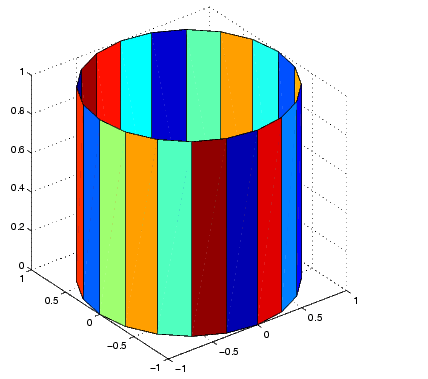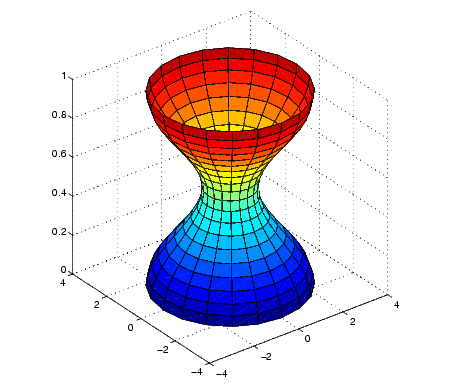MATLAB Function Referencecylinder

Generate cylinder

Syntax

• ```[X,Y,Z] = cylinder
[X,Y,Z] = cylinder(r)
[X,Y,Z] = cylinder(r,n)
cylinder(axes_handle,...)
cylinder(...)
```

Description

`cylinder` generates x-, y-, and z-coordinates of a unit cylinder. You can draw the cylindrical object using `surf` or `mesh`, or draw it immediately by not providing output arguments.

```[X,Y,Z] = cylinder ``` returns the x-, y-, and z-coordinates of a cylinder with a radius equal to `1`. The cylinder has 20 equally spaced points around its circumference.

```[X,Y,Z] = cylinder(r) ``` returns the x-, y-, and z-coordinates of a cylinder using `r` to define a profile curve. `cylinder` treats each element in `r` as a radius at equally spaced heights along the unit height of the cylinder. The cylinder has 20 equally spaced points around its circumference.

```[X,Y,Z] = cylinder(r,n) ``` returns the x-, y-, and z-coordinates of a cylinder based on the profile curve defined by vector `r`. The cylinder has `n` equally spaced points around its circumference.

```cylinder(axes_handle,...) ``` plots into the axes with handle `axes_handle` instead of the current axes (`gca`).

```cylinder(...), ``` with no output arguments, plots the cylinder using `surf`.

Remarks

`cylinder` treats its first argument as a profile curve. The resulting surface graphics object is generated by rotating the curve about the x-axis, and then aligning it with the z-axis.

Examples

Create a cylinder with randomly colored faces.

• ```cylinder
axis square
h = findobj('Type','surface');
set(h,'CData',rand(size(get(h,'CData'))))```

Generate a cylinder defined by the profile function `2+sin(t)`.

• ```t = 0:pi/10:2*pi;
[X,Y,Z] = cylinder(2+cos(t));
surf(X,Y,Z)
axis square```

See Also

`sphere`, `surf`

Polygons and Surfaces for related functionscustomverctrl daspect© 1994-2005 The MathWorks, Inc.